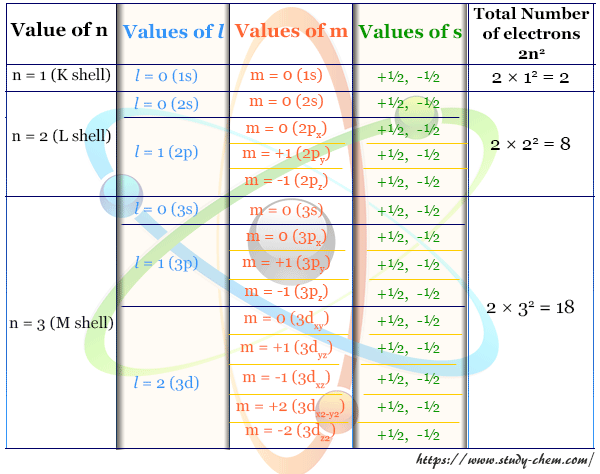Home Atom Quantum Number

# Quantum Number## What is Quantum Number?

Quantum number or principal (n), azimuthal (l), magnetic (m), and spin (s) quantum numbers in chemistry or physics describe the size, shape, and orientation of an orbital in an atom. Bohr model could not explain the fine structure of an atom. The appearance of several spectral lines by only one quantum number suggested by Bohr is not sufficient. The fact clearly indicated that the four quantum numbers needed to explain the various spectra of an atom. Therefore, the quantum numbers are the identification numbers for an individual electron of an atom which describe the position and energy of an electron in an atom.### Principal Quantum Number

The principal quantum number defines the energy level or principal shell to which an electron can stay. It is denoted by the letter n. It can only have a value from 1 to ∞, but 1 to 7 can be established. The capital letters K, L, M, N are also used to designate the values of n. For the hydrogen atom, the energy of an electron is fixed due to the fixed value of n.

#### How to find principal quantum number?

For multielectron atoms, the energy of each electron is dependent mostly on the value of the principal quantum number of the electron. As the value of n increases, the nucleus electron separation or the size of the orbital increases. The energy of the electron on the orbital is also raised.

### Azimuthal Quantum Numbers

Azimuthal or angular momentum or orbital quantum number is denoted by the letter l. It was introduced by Somerfield in his atomic model to describe the general geometric shape or the fine structure of the electron wave (orbital). The azimuthal quantum numbers (l) can have any values from 0 to (n-1) for a given value of n. Therefore, l = 0, 1, 2, … (n-2), (n-1).

#### How to find azimuthal quantum number?

The total number of different values of l is equal to n. Therefore, if n = 1, l = 0 (only one value); n = 2, l = 0, 1 (two values); n = 3, l = 0, 1, 2 (three values); n = 4, l = 0, 1, 2, 3 (four values). These values are define different types of orbitals like s (l=0), p (l = 1), d (l = 2) and f (l = 3).

### Magnetic quantum number

The third quantum number m defines the orientation of electron wave or orbital with respect to a given direction in presence of a strong electric or magnetic field. It does not affect the shape of the orbital or on the energy of an electron but uses to specify the sub-level of an atom.

#### How to find magnetic quantum number?

For a given value of l, m have any integral value between +l to -l. Therefore, m = 0, ±1, ±2, ±3, … ±l; where the total number of values of m equal to (2l +1). For p wave, l = 1, m has the values +1, 0, -1. These three orientations are expressed by the subscripts px, py, pz. For d-type electron wave, l = 2 and m = +2, +1, 0, -1, -2. Five m values describe the five orientations of space like dxy, dxz, dyz, dx2-y2, and dz2.

### Spin Quantum Numbers

When the spectral lines of hydrogen, lithium, sodium, and potassium are observed by the spectrometer with high resolving power, each line of the spectral series consisting of a pair of lines. These are known as doublets or double line fine structures. To account for these doublets, a spinning electron can have two possibilities. The electron can move either clockwise or anticlockwise. The two directions of spin define the fourth quantum number s with the values +1/2 or -1/2.